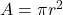What is the area of a circle is the radius is 8

Question

What is the area of a circle is the radius is 8

in progress 0
5 months 2021-08-19T08:56:45+00:00 2 Answers 0 views 0

1. Step-by-step explanation: To find the area of the circle,

start with the formula for the area of a circle, which is.

Notice that our circle has a radius of 8 units.

So plugging into the formula, we have (π)(8 units)².

Now, (8 units)² is equal to 8 units × 8 units or 64 units².

So we have 64π units² which is an exact answer.

2. When the radius is 8, the area would be 201.06

Area of circle = pi x radius² (pi x 8²)